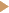# SQL Tutorial

Previous | Home  | Next

### SQL KeywordsIntroductionFROM, SELECT, WHERE, DISTINCTORDER BYAGGREGATE FUNCTIONSGROUP BYHAVINGINNER JOINLEFT JOIN

### ExamplesA structured approach to writing queriesExample 1Example 2Example 3Example 4Example 5Example 6
SQL course - Part 3

AGGREGATE FUNCTIONS

In certain cases, you are not actually interested in the data themselves but more in information about these data. For instance, you may want to know the number of rows in a certain table. A number of functions are available in SQL that help you to derive such information about the tables in your database. The following aggregate functions can be used:

 COUNT ( [column] ) counts the number of rows in a table SUM ( [column] ) adds all the numerical values in the specified column AVG ( [column] ) calculates the average of all the values in the specified column MIN ( [column] ) returns the minimum of all the numeric values in the specified column MAX ( [column] ) returns the maximum of all the numeric values in the specified column

When you use one of these functions, the result of the query will contain one row and one column only. This single cell contains the number that is the result of the statistical function that has been applied. An example is given below.

SELECT COUNT(*)
FROM CITY;

This query will return one row and one cell which contains the number of cities that are present in the table CITY.

COUNT() takes the name of a column as a paramter. This parameter should be included within parentheses. The function will count all the records for which the specified column is not empty. The best way to ensure that all the columns are selected is by using the asterisk (*) as a parameter, as in the example above. Each record in which any of the fields is not empty will be included in the count.

If you want to ensure that double values are not counted twice, you can of course use DISTINCT in order to get rid of these double values before you start counting. The following query counts the number of unique subject codes that have been used in the table TREASURE.

SELECT COUNT(DISTINCT SUBJECT)
FROM TREASURE ;

The query below selects the oldest year of birth in the table CREATOR:

SELECT MIN(YEAR_OF_BIRTH)
FROM CREATOR ;

Exercises

1. Select the year of the oldest treasure in the database
2. Count the number of different countries in the table TREASURE
3. Find the most recent year of death in the table PERSON

[ up  | next ]